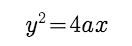# Let PQ be a focal chord of the parabola

Let PQ be a focal chord of the parabolaThe tangents to the parabola at P and Q meet at a point lying on the line y = 2x + a, a > 0.

1.Length of chord PQ is?

2.If chord PQ subtends an angle θ at the vertex of y2 = 4ax, then tanθ =

Devendra Yadav Maths 30 Apr, 2018 1955 views